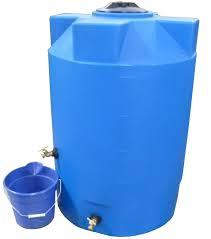# Temperature 3104

The tank has water with a volume of 300 liters and a temperature of 10 ° C. Add water at a temperature of 90 ° C until a temperature of 30 ° C is reached. How many liters of warmer water do I need to add?

x =  100

### Step-by-step explanation:

300·10+x·90=(300+x)·30

60x = 6000

x = 6000/60 = 100

x = 100

Our simple equation calculator calculates it.Did you find an error or inaccuracy? Feel free to write us. Thank you!

Tips for related online calculators
Do you have a linear equation or system of equations and looking for its solution? Or do you have a quadratic equation?